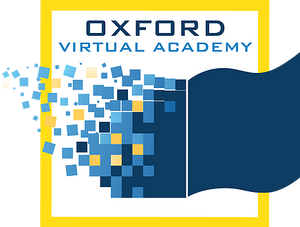# Math 4

This course expands on many third grade core mathematics concepts as well as introducing new concepts. These concepts include place value, reading and writing whole numbers, comparing numbers, ordering numbers, word problems with addition, subtraction, multiplication and division, rounding and estimating, counting and making change with money, factors and multiples, telling time and calculating elapsed time, measurement, 2-D and 3-D shapes, various graphs and diagrams, probability, adding, subtracting, multiplying, converting, and ordering fractions and decimals, completing function tables, and solving inequalities. They will also recognize, reproduce, extend, create and describe patterns. These topics include skills that are necessary to build a foundation in geometry, algebra, trigonometry, statistics, calculus, and real world problem solving.

* Prerequisites: None